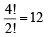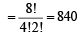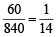JEE  >  True/False: Probability | JEE Advanced

# True/False: Probability | JEE Advanced - Notes | Study Maths 35 Years JEE Main & Advanced Past year Papers - JEE

 1 Crore+ students have signed up on EduRev. Have you?

Q. 1. If the letters of the word “Assassin” are written down at random in a row, the probability that no two S’s occur together is 1/35 (1983 - 1 Mark)

Ans. Sol. Let E be the event “No two S's occur together”.
A, A, I, N can be arranged inways

– A – A – I – N – Creating 5 places for 4 S. Out of 5 places 4 can be selected in C= 5 ways.

\ No two S’s occur together in = 12 × 5 = 60 ways Total no.of arranging all letters of word ‘assassin’\ Req. prob.  =\ Statement is False.

Q.2. If the probability for A to fail in an examination is 0.2 and that for B is 0.3, then the probability that either A or B fails is 0.5. (1989 - 1 Mark)

Ans. Sol.  P (A ∪  B) = P (A) + P (B) – P (A ∩ B)
= P (A) + P (B) – P (A) P (B)
[∵A and B are independent events] = 0.2 + 0.3 – 0.2 × 0.3 = 0.5 – 0.06 = 0.44 ≠ 0.5
\ The statement is false.

The document True/False: Probability | JEE Advanced - Notes | Study Maths 35 Years JEE Main & Advanced Past year Papers - JEE is a part of the JEE Course Maths 35 Years JEE Main & Advanced Past year Papers.
All you need of JEE at this link: JEE

## Maths 35 Years JEE Main & Advanced Past year Papers

132 docs|70 tests
 Use Code STAYHOME200 and get INR 200 additional OFF

## Maths 35 Years JEE Main & Advanced Past year Papers

132 docs|70 tests

Track your progress, build streaks, highlight & save important lessons and more!

,

,

,

,

,

,

,

,

,

,

,

,

,

,

,

,

,

,

,

,

,

;